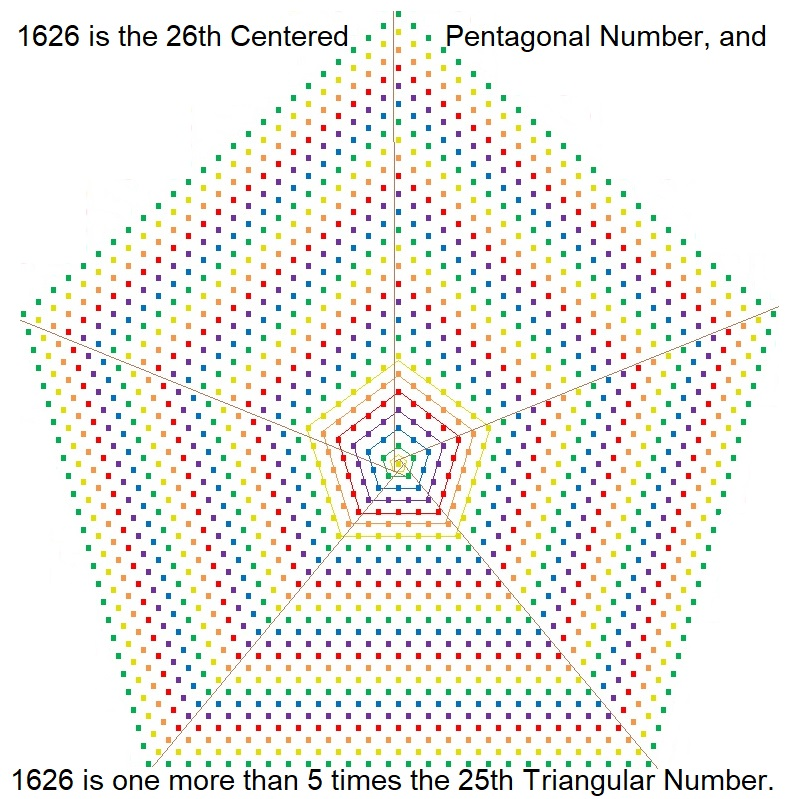# 1626 is a Centered Pentagonal Number

Contents

### Today’s Puzzle:

1626 is the 26th centered pentagonal number and it is also one more than 5 times the 25th triangular number.Today’s puzzle is for you to figure out why the following two expressions are equivalent. The first expression is the formula for the nth centered pentagonal number, and the second expression is one more than 5 times the formula for the nth triangular number.
(5n²-5n+2)/2, when n = 26, and
1 + 5n(n+1)/2, when n = 25.

### Factors of 1626:

• 1626 is a composite number.
• Prime factorization: 1626 = 2 × 3 × 271.
• 1626 has no exponents greater than 1 in its prime factorization, so √1626 cannot be simplified.
• The exponents in the prime factorization are 1, 1, and 1. Adding one to each exponent and multiplying we get (1 + 1)(1 + 1)(1 + 1) = 2 × 2 × 2 = 8. Therefore 1626 has exactly 8 factors.
• The factors of 1626 are outlined with their factor pair partners in the graphic below.### More about the Number 1626:

2(813)(1) = 1626 means that 2(813)(1), 813² – 1², 813² + 1² is a Pythagorean triple, and
2(271)(3) = 1626 means that 2(271)(3), 271² – 3², 271² + 3² is as well.

Calculate those expressions and you will have found the only two Pythagorean triples containing the number 1626.

This site uses Akismet to reduce spam. Learn how your comment data is processed.